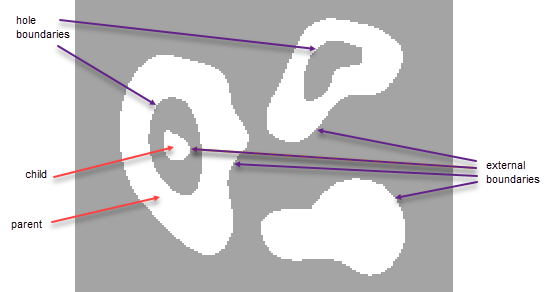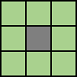# bwboundaries

## 语法

``B = bwboundaries(BW)``
``B = bwboundaries(BW,conn)``
``B = bwboundaries(___,options)``
``B = bwboundaries(___,Name=Value)``
``[B,L] = bwboundaries(___)``
``[B,L,n,A] = bwboundaries(___)``

## 说明

``B = bwboundaries(BW)` 跟踪二值图像 `BW` 中对象的外边界以及这些对象内部孔洞的边界。`bwboundaries` 还跟踪父对象完全包围的子对象的外边界和孔洞边界。该函数返回由边界像素位置组成的元胞数组 `B`。下图说明了这些分量。`
``B = bwboundaries(BW,conn)` 指定跟踪对象边界时要使用的 `conn` 连通性。`

``B = bwboundaries(___,options)` 跟踪对象的外边界，并通过将 `options` 设置为 `"holes"` 或 `"noholes"` 来指定是否包括孔洞的边界。`
``B = bwboundaries(___,Name=Value)` 使用名称-值参数 （自 R2023a 起）指定跟踪样式和返回的顶点坐标的顺序。`

``[B,L] = bwboundaries(___)` 还返回标签矩阵 `L`，该矩阵用于标记对象和孔洞。`

``[B,L,n,A] = bwboundaries(___)` 还返回 `n`（找到的对象数量）和 `A`（邻接矩阵）。`

## 示例

`I = imread('rice.png');`

`BW = imbinarize(I);`

```[B,L] = bwboundaries(BW,'noholes'); imshow(label2rgb(L, @jet, [.5 .5 .5])) hold on for k = 1:length(B) boundary = B{k}; plot(boundary(:,2), boundary(:,1), 'w', 'LineWidth', 2) end````BW = imread('blobs.png');`

`[B,L,N,A] = bwboundaries(BW);`

```imshow(BW); hold on; colors=['b' 'g' 'r' 'c' 'm' 'y']; for k=1:length(B), boundary = B{k}; cidx = mod(k,length(colors))+1; plot(boundary(:,2), boundary(:,1),... colors(cidx),'LineWidth',2); %randomize text position for better visibility rndRow = ceil(length(boundary)/(mod(rand*k,7)+1)); col = boundary(rndRow,2); row = boundary(rndRow,1); h = text(col+1, row-1, num2str(L(row,col))); set(h,'Color',colors(cidx),'FontSize',14,'FontWeight','bold'); end``````figure spy(A);````BW = imread('blobs.png');`

`[B,L,N] = bwboundaries(BW);`

```imshow(BW); hold on; for k=1:length(B), boundary = B{k}; if(k > N) plot(boundary(:,2), boundary(:,1), 'g','LineWidth',2); else plot(boundary(:,2), boundary(:,1), 'r','LineWidth',2); end end````BW = imread('blobs.png');`

```[B,L,N,A] = bwboundaries(BW); figure; imshow(BW); hold on; % Loop through object boundaries for k = 1:N % Boundary k is the parent of a hole if the k-th column % of the adjacency matrix A contains a non-zero element if (nnz(A(:,k)) > 0) boundary = B{k}; plot(boundary(:,2),... boundary(:,1),'r','LineWidth',2); % Loop through the children of boundary k for l = find(A(:,k))' boundary = B{l}; plot(boundary(:,2),... boundary(:,1),'g','LineWidth',2); end end end```## 输入参数

`4``8``"holes"`

`"noholes"`

### 名称-值参数

`"pixelcenter"`

`"pixeledge"`

`"yx"`

`"xy"`

## 输出参数

```enclosing_boundary = find(A(k,:)); enclosed_boundaries = find(A(:,k));```

## 算法

`bwboundaries` 函数实现由雅可比的终止条件修正的 Moore 邻点跟踪算法。此函数基于 `boundaries` 函数，后者出现在 Digital Image Processing Using MATLAB 第一版中。

 Gonzalez, R. C., R. E. Woods, and S. L. Eddins, Digital Image Processing Using MATLAB, New Jersey, Pearson Prentice Hall, 2004.